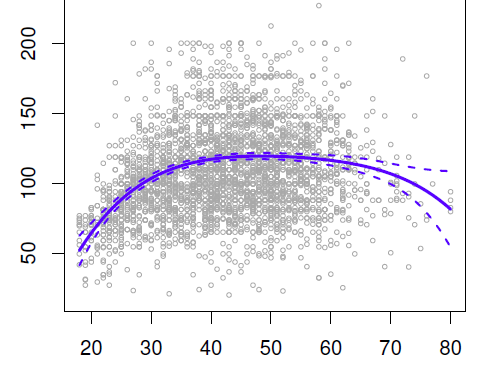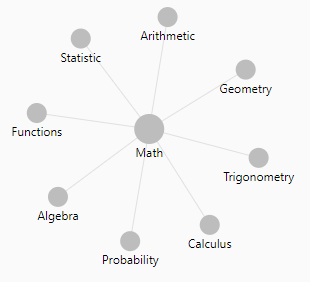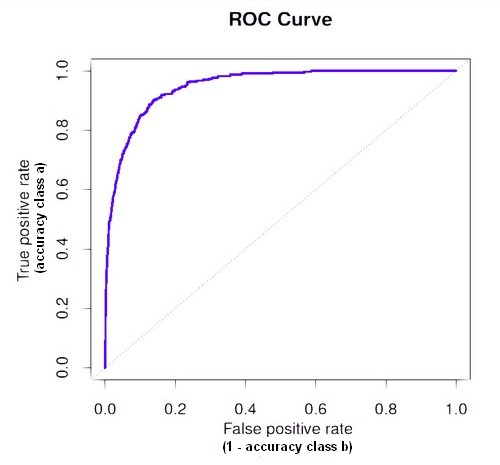# Statistics - Probability (of an event) / Likelihood

The probability of an event is the subjective chance that it will happen.

The laws of probability are heavily build with the random notion.

A probability is a non-negative number between 0 and 100%.

If you add all the possible event on the x axis, you get a probability distribution.

To verify the the result, you can simulate the event through the creation of a script.

## Event

### One

$$\text{Probability of event A} = \frac{\text{Number of possible outcomes fitting our criteria}}{\text{Total number of possible outcomes}} = P(A)$$

$$\text{Odds of event} = \frac{\text{Number of successes}}{\text{Number of non-successes}}$$

If the probability of an event is 3/5, the odds are 3/2.

### Independent

If the events are independent, we can multiply each probability. Two events are independent when the outcome of the first event does not influence the outcome of the second event.

$$P(A \text{ and } B) = P(A) . P(B)$$

### Dependent

Two events are dependent when the outcome of the first event influences the outcome of the second event. see Statistics - Bayes’ Theorem (Probability)

$$P(A \text{ and } B) = P(A) . P(B \text{ after } A)$$

Example: Probability to choose 2 red cards in a deck of 52 cards. $$P(\text{Red card}) = \frac{26}{52}$$

$$P(\text{2 Red cards}) = \frac{26}{52} . \frac{25}{51}$$

### Exclusive

Exclusive events cannot happen at the same time.

$$P(A \text{ or } B) = P(A) + P(B)$$

Example:

• wheel of fortune, only one field can be chosen. The chance to go the field 1 or 2.
• the chance that two persons will stay at the same hotel in a set of 3 hotels (A, B, C)

$$P(A \text{ or } B \text{ or } C) = P(A) + P(B) + P(C) = (\frac{1}{3}.\frac{1}{3}) + (\frac{1}{3}.\frac{1}{3}) + (\frac{1}{3}.\frac{1}{3}) = \frac{3}{9} = = \frac{1}{3}$$

### Inclusive

Inclusive events can happen at the same time.

$$P(A \text{ or } B) = P(A) + P(B) - P(A \text{ and } B)$$

Example: What is the probability of drawing a black card or a ten in a deck of cards? This probability is inclusive because you can have in one event the two possibles outcomes.

• Event A: drawing a black card: 26 outcomes
• Event B: drawing a ten card: 4 outcomes
• Event A and B: drawing a ten black card: 2 outcomes
• Total of possible outcomes: 52 cards

$$P(A \text{ or } B) = \frac{26}{52} + \frac{4}{52} - \frac{2}{52}$$

## Category

### Frequency

For the frequentist a hypothesis is a proposition (which must be either true or false), so that the frequentist probability of a hypothesis is either one or zero.

### Evidential

In Bayesian statistics, a probability can be assigned to a hypothesis that can differ from 0 or 1 if the true value is uncertain.

### Propensity

A coin is tossed repeatedly many times, in such a way that its probability of landing heads is the same on each toss, and the outcomes are probabilistically independent, then the relative frequency of heads will (with high probability) be close to the probability of heads on each single toss. This law suggests that stable long-run frequencies are a manifestation of invariant single-case probabilities.

Frequentists are unable to take this approach, since relative frequencies do not exist for single tosses of a coin, but only for large ensembles or collectives. Hence, these single-case probabilities are known as propensities or chances.

## Bayesian and Frequentist Concepts

Differences between Bayesian and Frequentist Concepts

Frequentist Bayesian
Data are a repeatable random sample (there is a frequency) Data are observed from the realized sample
Underlying parameters remain constant during this repeatable process Parameters are unknown and described probabilistically
Parameters are fixed Data are fixed

## Documentation / Reference

Discover MoreData Mining - (Global) Polynomial Regression (Degree)

polynomials regression Although polynomials are easy to think of, splines are much better behaved and more local. With polynomial regression, you create new variables that are just transformations...Mathematics - Factorial

The factorial of a non-negative integer x is equal to the product of all integers less than or equal to x and greater than zero. For example: factorial(4) would equal 4 3 2 1, which is 24. In factorial,...Mathematics - Probability distribution function

A Probability distribution function is a function that is used that specify relative likelihood (probability) of different outcomes of a single experiment. It assigns a probability (a nonnegative number)...Statistics - (Confidence|likelihood) (Prediction probabilities|Probability classification)

Prediction probabilities are also known as: confidence (How confident can I be of this prediction?). or likelihood: (How likely is this prediction to be true?) They gives the probability of a predicted...Statistics - (Base rate fallacy|Bonferroni's principle)

Every accurate (model|test) can be useless as detection tools if the studied case is sufficiently rare among the general population. The data model will produce too many false positives or false negatives....Statistics - (Normal|Gaussian) Distribution - Bell Curve

A normal distribution is one of underlying assumptions of a lot of statistical procedures. In nature, every outcome that depends on the sum of many independent events will approximate the Gaussian distribution...Statistics - Bayes’ Theorem (Probability)

Bayesian probability is one of the different interpretations of the concept of probability and belongs to the category of evidential probabilities. In the Bayesian view, a probability is assigned to a...Statistics - Central limit theorem (CLT)

The Central_limit_theoremcentral limit theorem (CLT) is a probability theorem (unofficial sovereign) It establishes that when: random variables (independent) (estimate of a random process) are added...Statistics - ROC Plot and Area under the curve (AUC)

The Area Under Curve (AUC) metric measures the performance of a binary classification. In a regression classification for a two-class problem using a probability algorithm, you will capture the probability...Statistics - log-likelihood function (cross-entropy)

The “log-likelihood function” is a probabilistic function. The “log-likelihood function” is also referred to as the cross-entropy